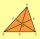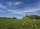# Prove

Prove that k1 and k2 is the equations of two circles. Find the equation of the line that passes through the centers of these circles.

k1: x2+y2+2x+4y+1=0
k2: x2+y2-8x+6y+9=0

Result

p = (Correct answer is: p=x+5y+11=0)#### Solution:Leave us a comment of example and its solution (i.e. if it is still somewhat unclear...):

Showing 0 comments:Be the first to comment!#### To solve this example are needed these knowledge from mathematics:

For Basic calculations in analytic geometry is helpful line slope calculator. From coordinates of two points in the plane it calculate slope, normal and parametric line equation(s), slope, directional angle, direction vector, the length of segment, intersections the coordinate axes etc. Looking for help with calculating roots of a quadratic equation? Do you have a linear equation or system of equations and looking for its solution? Or do you have quadratic equation? Pythagorean theorem is the base for the right triangle calculator. See also our trigonometric triangle calculator.

## Next similar examples:

1. Theorem proveWe want to prove the sentense: If the natural number n is divisible by six, then n is divisible by three. From what assumption we started?
2. RootsDetermine the quadratic equation absolute coefficient q, that the equation has a real double root and the root x calculate: ?
3. CircleCircle touch two parallel lines p and q; and its center lies on a line a, which is secant of lines p and q. Write the equation of circle and determine the coordinates of the center and radius. p: x-10 = 0 q: -x-19 = 0 a: 9x-4y+5 = 0
4. RTriangle 17The hypotenuse of a right triangle is 17 cm. If you decrease both two legs by 3 cm you will reduce the hypotenuse by 4 cm. Determine the length of this legs.
5. RT and circlesSolve right triangle if the radius of inscribed circle is r=9 and radius of circumscribed circle is R=23.
6. On lineOn line p: x = 4 + t, y = 3 + 2t, t is R, find point C, which has the same distance from points A [1,2] and B [-1,0].
7. CenterIn the triangle ABC is point D[1,-2,6], which is the center of the |BC| and point G[8,1,-3], which is the center of gravity of the triangle. Find the coordinates of the vertex A[x,y,z].
8. Quadratic equationFind the roots of the quadratic equation: 3x2-4x + (-4) = 0.
9. VariableFind variable P: PP plus P x P plus P = 160
10. Distance problem 2A=(x,2x) B=(2x,1) Distance AB=√2, find value of x
11. CircleFrom the equation of a circle: ? Calculate the coordinates of the center of the circle S[x0, y0] and radius of the circle r.
12. EquationEquation ? has one root x1 = 8. Determine the coefficient b and the second root x2.
13. CathetiThe hypotenuse of a right triangle is 41 and the sum of legs is 49. Calculate the length of its legs.
14. DiscriminantDetermine the discriminant of the equation: ?
15. Solve 3Solve quadratic equation: (6n+1) (4n-1) = 3n2
16. The fenceI'm building a fence. Late is rounded up in semicircle. The tops of late in the field between the columns are to copy an imaginary circle. The tip of the first and last lath in the field is a circle whose radius is unknown. The length of the circle chord i
17. Triangle IRTIn isosceles right triangle ABC with right angle at vertex C is coordinates: A (-1, 2); C (-5, -2) Calculate the length of segment AB.